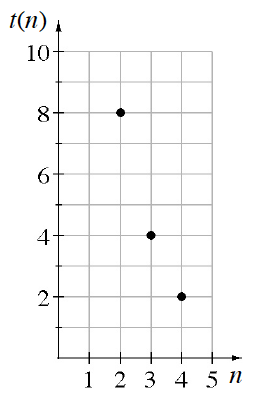### Home > CC3MN > Chapter 10 > Lesson 10.2.3 > Problem10-73

10-73.

Write an explicit equation for the sequence graphed at right.

The multiplier for this graph is $\frac{1}{2}$

Work backward to find term $0$, or the starting value.

Use the starting value and the multiplier to write the equation.

$t(n)=32\left( \frac{1}{2} \right)^n$